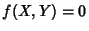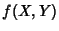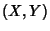## Algebraic Curve

An algebraic curve over a Fieldis an equation, whereis a Polynomial inandwith Coefficients in. A nonsingular algebraic curve is an algebraic curve overwhich has no Singular Points over. A point on an algebraic curve is simply a solution of the equation of the curve. A-Rational Point is a pointon the curve, whereandare in the Field.